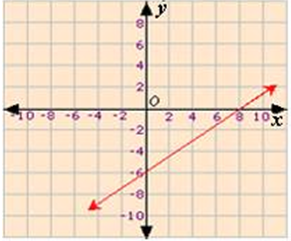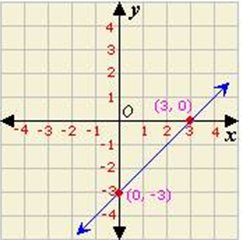## Definition Of Y-Intercept

The y-coordinate of the point where a line intersects the y-axis is the y-intercept of that line.

The x-coordinate of the point where a line intersects the y-axis is 0. So, the y-intercept of a line can also be found by substituting x = 0 in the equation of the line.

### Examples of Y-InterceptThe y-intercept of the line shown is -6.

### Solved Examples on Y-Intercept

#### Ques: Find the y-intercept of the line shown in the graph.A. - 3
B. 0
C. 3
D. 1